# Use Formula taking reference of a particular cell across any time period• Say, I have 10 periods: 6 periods in past and 4 periods in future (i.e. the 7th period is the current period).
• I calculate the mean of the first 6 periods (past periods) and store the same in the current period (say line item "Mean").
• Now, I want to calculate the deviation of the actuals (values in the past) from the mean value.
• But mean value is present only in the current period.

How can I do 'Actuals - Current period mean value' across each time period in the past? The excel equivalent of this is using the dollar sign for a cell value (so the cell value of mean is fixed).

Thanks,

Regards,

Guru

Tagged:

•Try it this way;

1. Change your current mean formula to MOVINGSUM(Historical Sales, -(Cumulated History Periods), -1, AVERAGE). It's the same formula without the IF condition. Call this 'Mean Across Time'.

2. Now another line item, without any dimensions, with the formula: PERIOD(CURRENTPERIODSTART()). Call it 'Current Period'

3. Now your final line item which you will refer for other calculations when you need mean; have the dimension of time and the formula will be:

Mean Across Time[LOOKUP: Current Period]

Let me know if this works

Regards,

Anirudh

•Hi @GuruAP ,

I tried to simulate your requirement here to calculate the Mean, standard deviation, Lower Bound Outlier and Upper Bound Outlier based on actuals. for that I have created three Modules called Data Module, Stats Module, Time Period Module. Stats Module is not using time dimension. Current Period is W/c 5 Jan 20.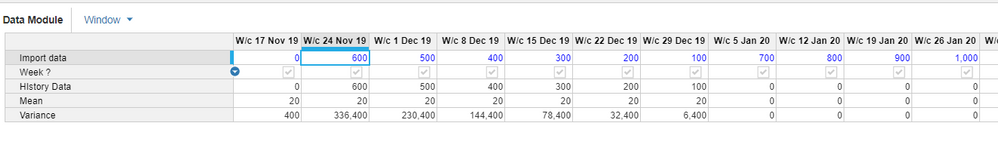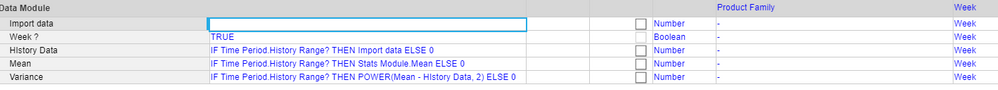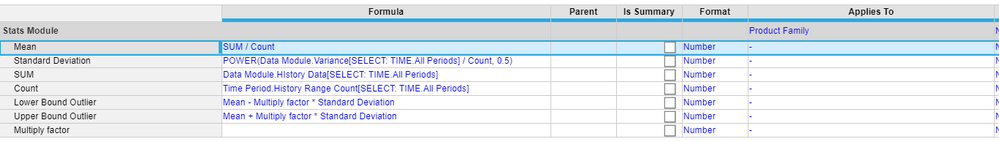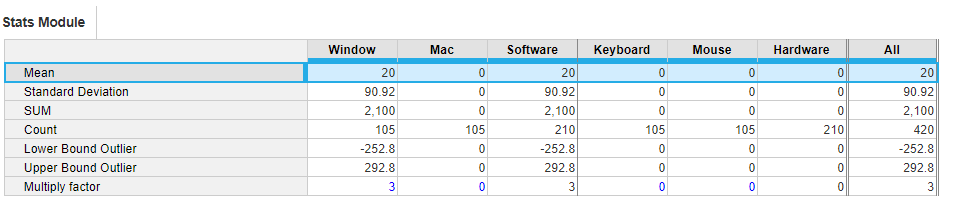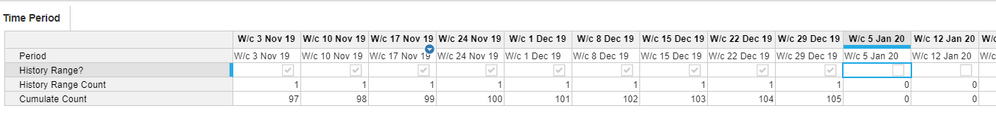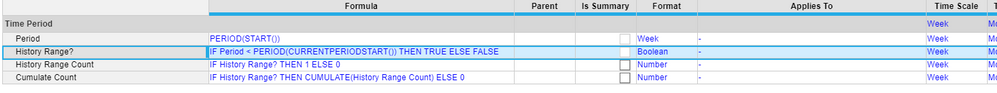Thanks,

•Great question Guru,

The \$ sign equivalent in Anaplan is to remove the particular dimension in the line item. So what you have to do is calculate your current period value in a line item and then remove the time dimension from that line item (refer screenshot)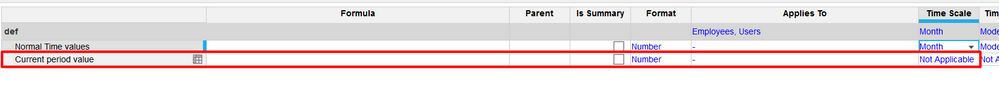Subsequently when you refer this line item to others, it will subtract only the current period value

Let me know if you need help setting this up, you will need to do it using a lookup formula

Regards,

Anirudh

•Guru-

By using TIME.'Current Period' in a formula it will always pull in the current period based on the model time settings. You could either use this with a Select Statement: Mean Value[SELECT: TIME.'Current Period'] -or- by putting a new line item in a SYSTEMS Module for Current Period with a formula of TIME.'Current Period'. Then your formula would read: Mean Value[LOOKUP:Current Period Line item]

Hope this helps,

Jason

•Hi,

As long as Planual recommends to not hardcode any values directly in the formulas and make the calculation scalable, the best way would be to get the Mean calculated properly first.

Do it on a separate line item with required dimensions only.

If you always need Mean be calculated as a sum of 6 months, then as said earlier - you do not need the timescale for calc. Do that 6 months sum once and consider that a fixed value to linked to any certain month for further reference

If Mean should be calculated differently for every month within a year and you know the exact rule on how to calc it - use timescale as a dimension and design the scalable formula for calculation.

For example you can get values for only actualised months via IF formula and put 0 for all remaining periods. In a next step you can refer SELECT: TIME.ALL PERIODS or SELECT: TIME.YTD  to get the sum of all actualised periods and use that value as a denominator in you original calculation. There are also some different techniques available if you work within few years - to use LOOKUP by Year for example, but I hope something simple can work in your case

•If you intend to use the MEAN calculation across multiple modules then I would create a new module and remove the time dimension.

TIMESUM formula can be used to calculation the mean AVERAGE.

The formulas in this new module refer to line items which should be created in a current time properties module. If you have already created such a module to hold all your current time properties add the following two line items. If you have yet to build this module do so now as it will prove invaluable as the model develops. This new time properties module should contain no dimensions and only the following line items.

Module name - Current Time Properties

1. Line item name - Current Period, Format - Time period months, formula - PERIOD(CURRENTPERIODEND())

2. Line item name - Current Year, Format - Time period years, formula - Current Period

3. Line item name - Current Year Start, Format - Time period months, formula - PERIOD(START(Current Year))

In new module create the following three line items;

Module name - MEAN Calculation

1. Name - Mean, Format - Number, Formula - TIMESUM(source module.source line item, Current Time Properties.Current Period, Current Time Properties.Current Year Start, AVERAGE)

Notice how I have used the current period and current year start line items from the time properties module to inform the function over which periods to calculate the MEAN. As the current period is updated so will the range over which the calculation operates. Therefore, it updates dynamically as the year progresses.

Now rather than using subsidiary view to hold the MEAN calculation you can now reference it throughout you model if the circumstances required are the same. This ensures that you are calculating once and referencing many times a key point in managing the size and performance of your models.

This approach also does not require you to use any LOOKUP functions which can frustrate summary options as the only option available is SUM. This is likely to not be appropriate if the calculation is to incorporated into a complex function downstream where the summarising across time and up hierarchies means the FORMULA summary option is required.

Chris

•Hi Anirudh - We want to normalize the sales history by calculating the outliers. The outliers we decided will be:
Mean - (Factor) * (Standard Deviation)
Mean + (Factor) * (Standard Deviation)
For mean, I did the following on the sales history:
IF PERIOD(START()) = TIME.'Current Period' THEN MOVINGSUM(Historical Sales, -(Cumulated History Periods), -1, AVERAGE) ELSE 0
With this, I have the MEAN calculated in the current period.
But for standard deviation, I need to have the deviation to calculate each sales history in each time bucket with that of the mean.
When I remove the time scale, it says START() function is used.
So wondering how I can achieve this.
Thanks,
Regards - Guru